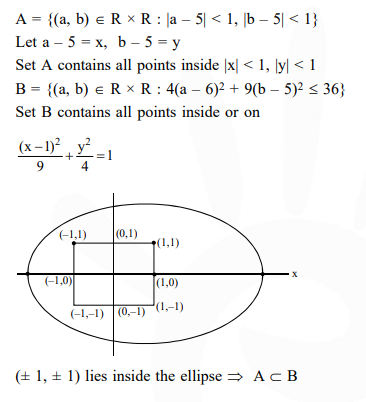# Two sets A and B are as under

Question:

Two sets $A$ and $B$ are as under

$\mathrm{A}=\{(\mathrm{a}, \mathrm{b}) \in \mathrm{R} \times \mathrm{R}:|\mathrm{a}-5|<1$ and $|\mathrm{b}-5|<1\}$

$\mathrm{B}=\left\{(\mathrm{a}, \mathrm{b}) \in \mathrm{R} \times \mathrm{R}: 4(\mathrm{a}-6)^{2}+9(\mathrm{~b}-5)^{2} \leq 36\right\} .$ Then :-

1. $A \subset B$

2. $A \cap B=\phi($ an empty set $)$

3. neither $\mathrm{A} \subset \mathrm{B}$ nor $\mathrm{B} \subset \mathrm{A}$

4. $\mathrm{B} \subset \mathrm{A}$

Correct Option: 1

Solution: